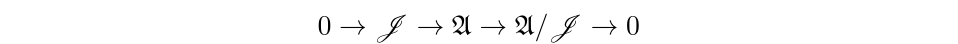# Operator Algebras Modeling Dynamics of Frames

For a Hilbert space $\mathscr{H}$, $y \in \mathscr{H}$ and $T \in \mathscr{B}(\mathscr{H})$, the equation \begin{equation} T x = y \end{equation} has a solution whenever $T$ is left invertible. For any left inverse $L$ of $T$, $x = L y$ is a solution. More generally, if $T$ has closed range, $T$ has a pseudo left inverse. Concretely, if $T \in \mathscr{B}(\mathscr{H})$ has closed range, restricting to $\ker(T)^\perp$ we obtain a new operator that is left invertible. Picking a left inverse $L$ for $T\mid_{\ker(T)^\perp}$, we find that $x = L y$ is a solution.

In most applications, there is a canonical choice for the left inverse $L$. Generally, this is the Moore-Penrose inverse, denoted $T^\dagger$. It is the unique left inverse such that $\ker(T^\dagger) = \mbox{ran}(T)^\perp$. To construct $T^\dagger$, one considers $T$ mapping $\ker(T)^\perp$ to $\mbox{ran}(T)$. From this perspective, $T$ is bijective, and thus boundedly invertible by the open mapping theorem. The unique inverse, once extended by zero, is $T^\dagger$. For instance, if $T$ is an isometry then $T^\dagger = T^*$, the adjoint of $T$.

One subject where closed range operators are frequently used is frame theory. A sequence {$f_n$} in a Hilbert space $\mathscr{H}$ is said to be a frame if there exists constants $0< A \leq B$ such that \begin{equation} A ||x||^2 \leq \sum_n | \langle x, f_n \rangle |^2 \leq B ||x||^2 \end{equation} for all $x \in \mathscr{H}$.

Associated to each Hilbert space frame {$f_n$} is a (canonical) dual frame {$g_n$}. Using this dual frame, one can reconstruct elements $f$ of the Hilbert space $\mathscr{H}$ in an analogous way to orthonormal basis:

\begin{equation} f = \sum_{n \geq 1} \langle f, g_n \rangle f_n \end{equation}

It is easy to see that orthonormal bases are frames, but not all frames need be orthogonal, norm one, or even contain a unique set of elements. A frame does not enforce the rigidity of inner products that an orthonormal basis does - allowing for variation between individual frame elements (rather than just 0 or 1). The flexibility of the definition has engendered applications across signal processing and harmonic analysis. For instance, frames may be constructed for particular features of a problem that are precluded from orthogonal bases due to their requirement for linear independence. Further, this extra redundancy helps to protect signals from degradation, ensuring that the effects of erasures are minimized. The looseness of the structure allows one to construct the analog of frames for structures that don’t necessarily come equipped with suitable generalization of an orthonormal basis. Indeed, certain classes of Hilbert C*-Modules and Banach spaces posses frames.

Many questions concerning Hilbert space frames are inexorably tied to questions of closed ranged and left invertible operators. All frames for closed subspaces of a Hilbert $\mathscr{H}$ spaces arise as the application of a closed range operator $T$ on a frame for $\mathscr{H}$. Properties of frames, such as rigidity and perturbation results also depend on the analysis of closed range operators. With this groundwork of closed ranged operators and frame theory laid, we now describe our interest in generating concrete operator algebras.

A well-studied class of operator algebras are C-algebras generated by partial isometries. For example graph algebras, C-algebras generated partial isometries subject to constraints of a directed graph, reside here.

Representations of graph C*-algebras arising from a directed graph may be described as follows. Given a directed graph, choose a Hilbert space for each vertex of the graph (with suitable dimension depending on loops of the graph). After direct summing each of these spaces together, we obtain a Hilbert space $\mathscr{H}$ where each vertex corresponds to an orthogonal summand of $\mathscr{H}$. By choosing orthonormal sequences for each of these closed, orthogonal subspaces of $\mathscr{H}$, we then pick partial isometries that map one summand to another, subject to the Cuntz-Krieger relations.

The partial isometric generators of concrete graph algebras preserve orthonormal basis (that is, up to the kernels of the partial isometries). The representation of the graph C-algebra can be viewed as encoding the dynamics of walks on the graph. A natural extension of these concrete graph C-algebras is one that encodes the dynamics of a frame.

One arrives at a such an extension by replacing partial isometries and their adjoints with closed ranged operators and their Moore-Penrose inverses. As discussed above, the closed range operators preserve frame theoretic quantities. In the isometric case, the Moore-Penrose inverse is the adjoint. Hence, such operator algebras reduce to the C-algebraic case when the generators are isometric. Therefore, this class of operator algebras is the natural generalization of concrete graph C-algebras that integrates frame theory. This leads us to the following general program:

Given a set of operators with closed range and their Moore-Penrose inverses construct the norm-closed algebra subject to the constraints of a directed graph. What is the structure of these algebras?

## Publications

. Operator Algebras Generated by Left Invertibles. Thesis, 2019.

## Talks

Operator Algebras Generated by Left Invertibles
May 25, 2020 12:00 AM
Operator Algebras Generated by Left Invertibles
Mar 13, 2019 12:00 AM